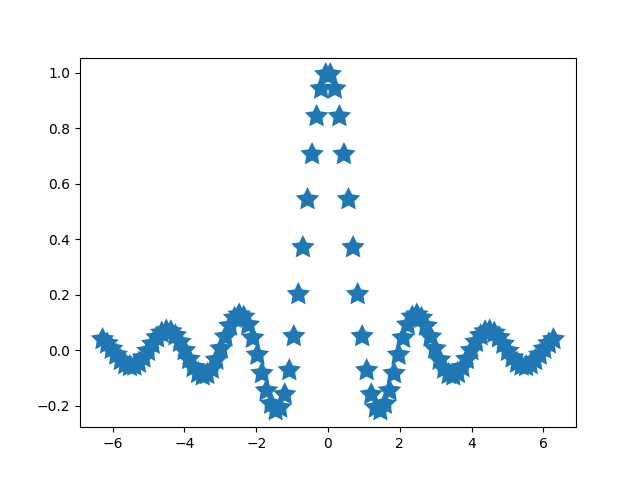# Autoscaling¶

The limits on an axis can be set manually (e.g. `ax.set_xlim(xmin, xmax)`) or Matplotlib can set them automatically based on the data already on the axes. There are a number of options to this autoscaling behaviour, discussed below.

We will start with a simple line plot showing that autoscaling extends the axis limits 5% beyond the data limits (-2π, 2π).

```import numpy as np
import matplotlib as mpl
import matplotlib.pyplot as plt

x = np.linspace(-2 * np.pi, 2 * np.pi, 100)
y = np.sinc(x)

fig, ax = plt.subplots()
ax.plot(x, y)
```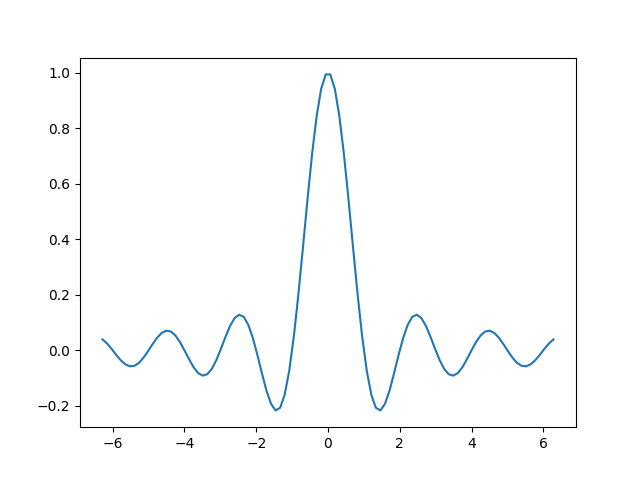Out:

```[<matplotlib.lines.Line2D object at 0x7fd1db64e130>]
```

## Margins¶

The default margin around the data limits is 5%:

```ax.margins()
```

Out:

```(0.05, 0.05)
```

The margins can be made larger using `margins`:

```fig, ax = plt.subplots()
ax.plot(x, y)
ax.margins(0.2, 0.2)
```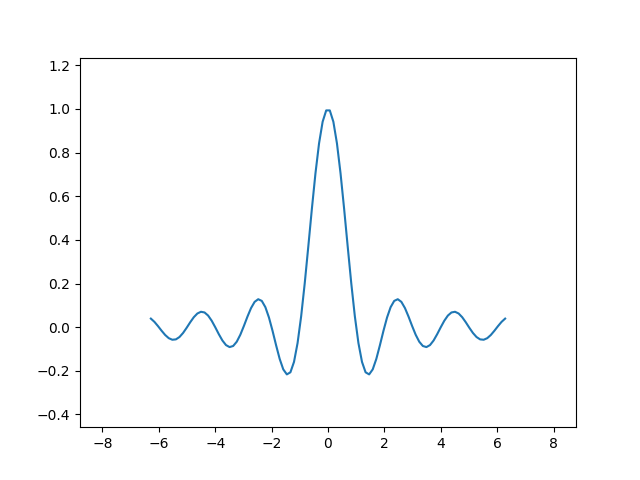In general, margins can be in the range (-0.5, ∞), where negative margins set the axes limits to a subrange of the data range, i.e. they clip data. Using a single number for margins affects both axes, a single margin can be customized using keyword arguments `x` or `y`, but positional and keyword interface cannot be combined.

```fig, ax = plt.subplots()
ax.plot(x, y)
ax.margins(y=-0.2)
```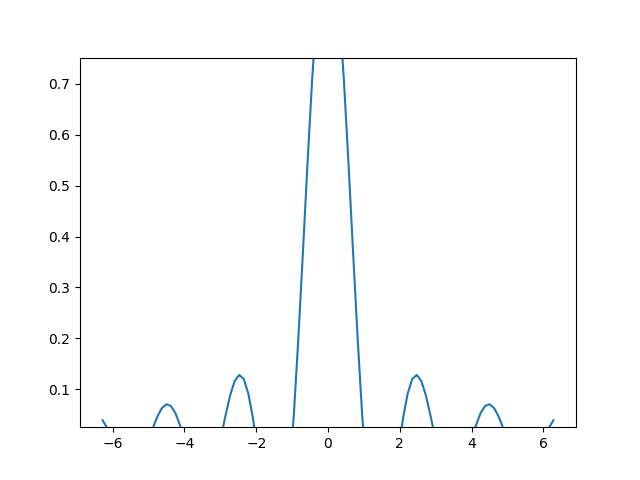## Sticky edges¶

There are plot elements (`Artist`s) that are usually used without margins. For example false-color images (e.g. created with `Axes.imshow`) are not considered in the margins calculation.

```xx, yy = np.meshgrid(x, x)
zz = np.sinc(np.sqrt((xx - 1)**2 + (yy - 1)**2))

fig, ax = plt.subplots(ncols=2, figsize=(12, 8))
ax.imshow(zz)
ax.set_title("default margins")
ax.imshow(zz)
ax.margins(0.2)
ax.set_title("margins(0.2)")
```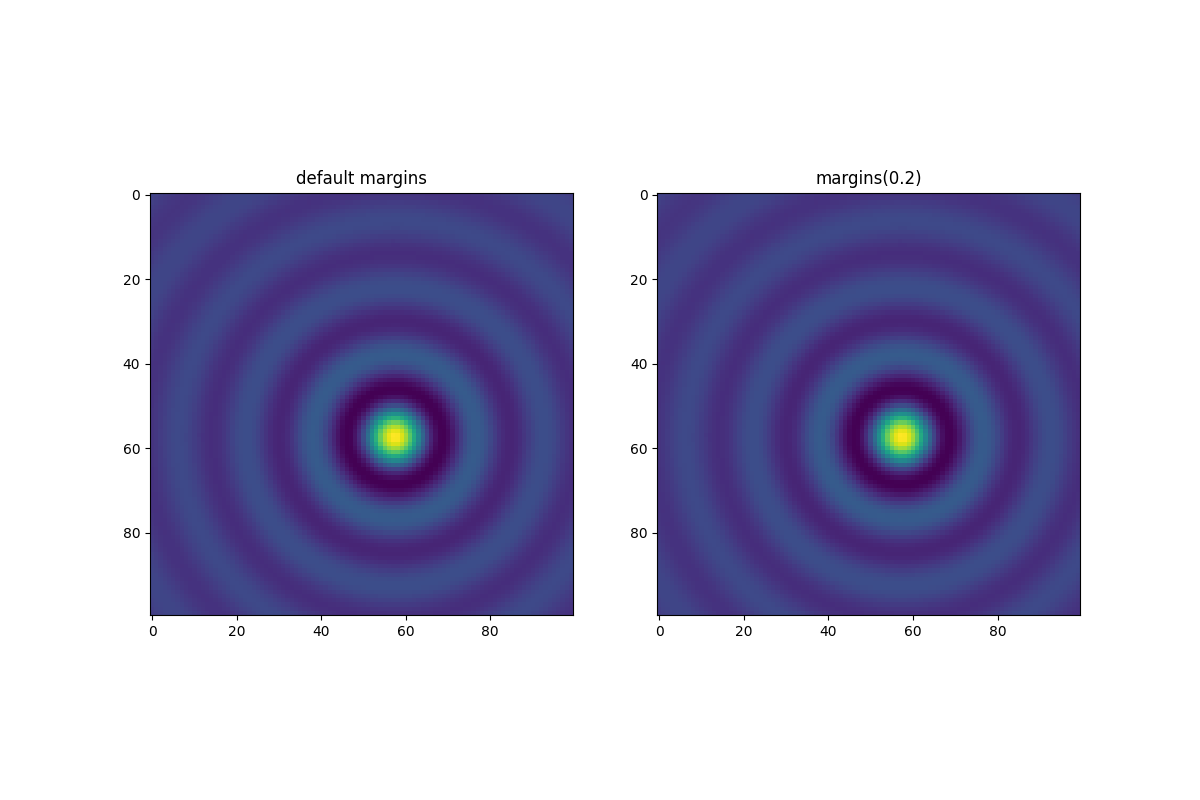Out:

```Text(0.5, 1.0, 'margins(0.2)')
```

This override of margins is determined by "sticky edges", a property of `Artist` class that can suppress adding margins to axis limits. The effect of sticky edges can be disabled on an Axes by changing `use_sticky_edges`. Artists have a property `Artist.sticky_edges`, and the values of sticky edges can be changed by writing to `Artist.sticky_edges.x` or `Artist.sticky_edges.y`.

The following example shows how overriding works and when it is needed.

```fig, ax = plt.subplots(ncols=3, figsize=(16, 10))
ax.imshow(zz)
ax.margins(0.2)
ax.set_title("default use_sticky_edges\nmargins(0.2)")
ax.imshow(zz)
ax.margins(0.2)
ax.use_sticky_edges = False
ax.set_title("use_sticky_edges=False\nmargins(0.2)")
ax.imshow(zz)
ax.margins(-0.2)
ax.set_title("default use_sticky_edges\nmargins(-0.2)")
```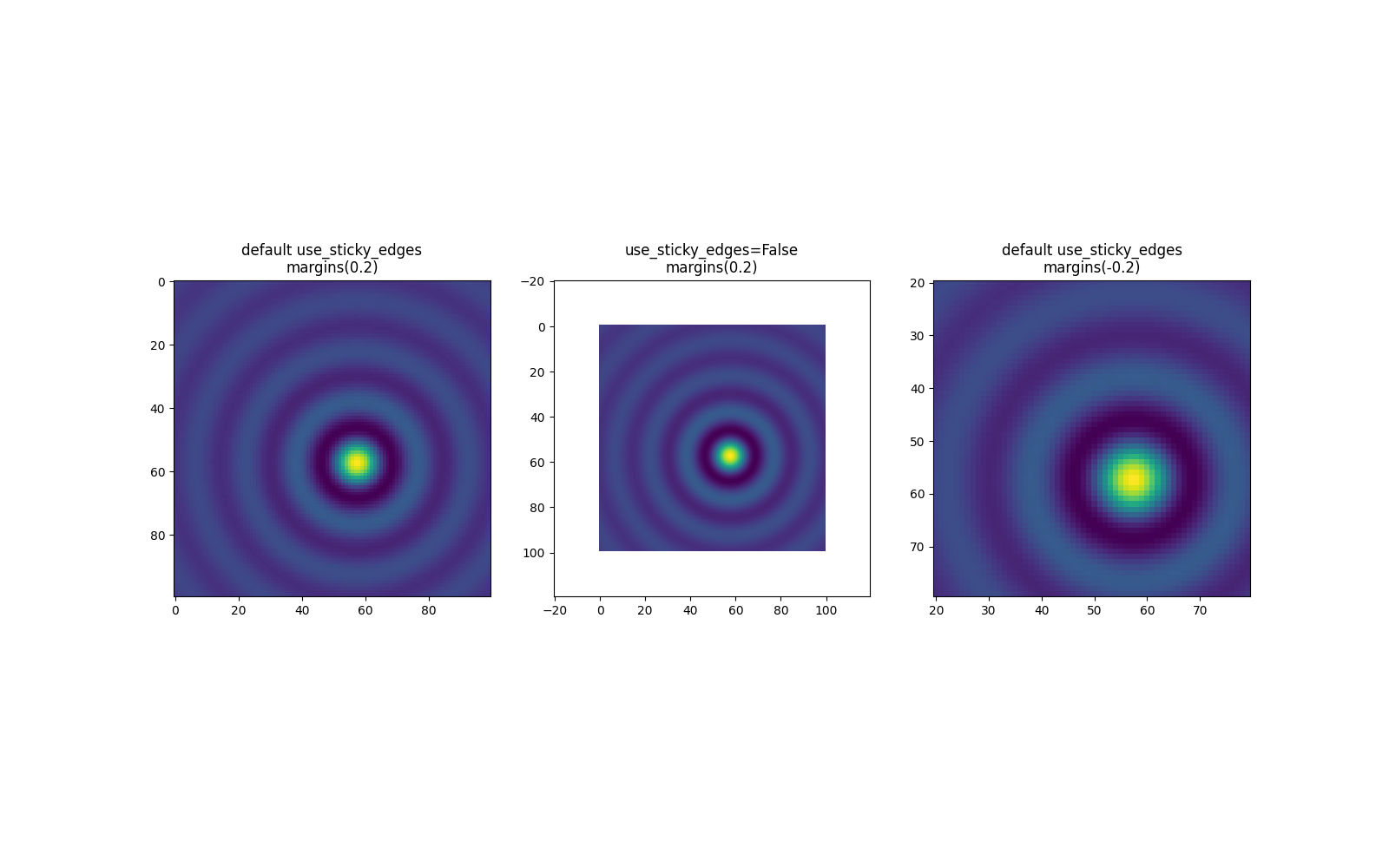Out:

```Text(0.5, 1.0, 'default use_sticky_edges\nmargins(-0.2)')
```

We can see that setting `use_sticky_edges` to False renders the image with requested margins.

While sticky edges don't increase the axis limits through extra margins, negative margins are still taken into account. This can be seen in the reduced limits of the third image.

## Controlling autoscale¶

By default, the limits are recalculated every time you add a new curve to the plot:

```fig, ax = plt.subplots(ncols=2, figsize=(12, 8))
ax.plot(x, y)
ax.set_title("Single curve")
ax.plot(x, y)
ax.plot(x * 2.0, y)
ax.set_title("Two curves")
```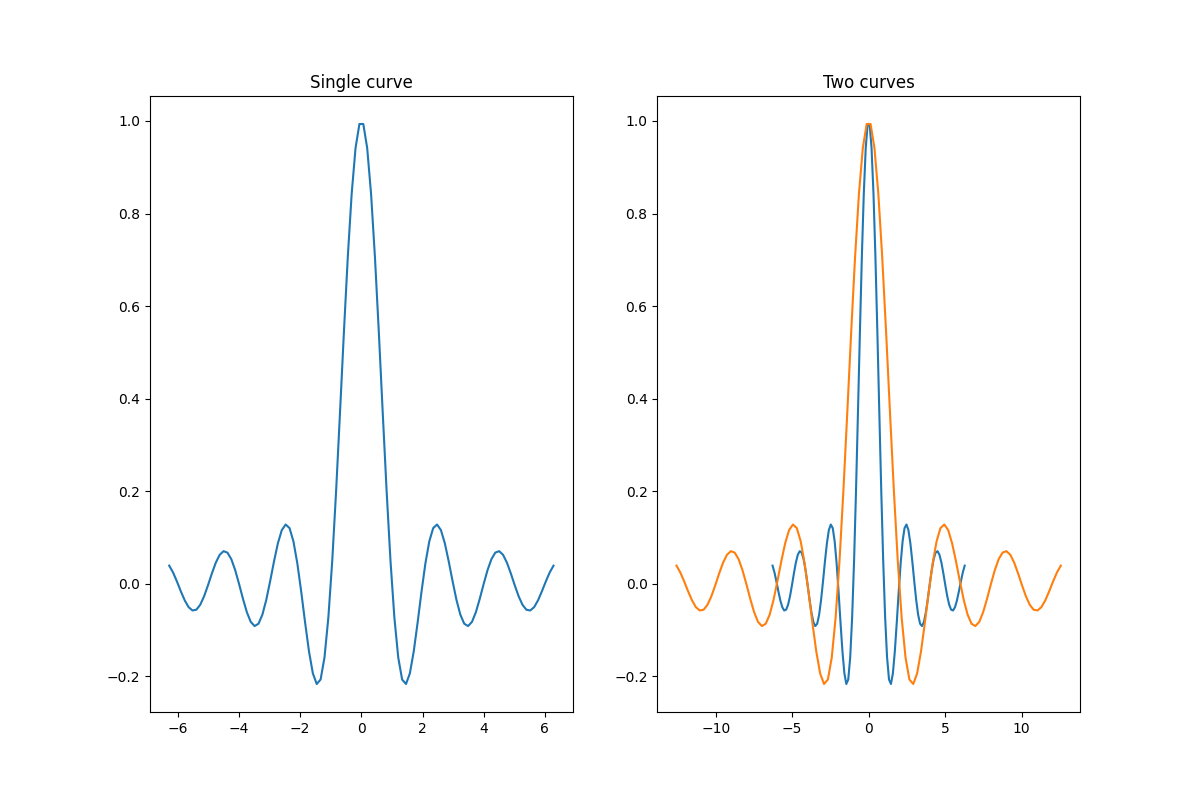Out:

```Text(0.5, 1.0, 'Two curves')
```

However, there are cases when you don't want to automatically adjust the viewport to new data.

One way to disable autoscaling is to manually set the axis limit. Let's say that we want to see only a part of the data in greater detail. Setting the `xlim` persists even if we add more curves to the data. To recalculate the new limits calling `Axes.autoscale` will toggle the functionality manually.

```fig, ax = plt.subplots(ncols=2, figsize=(12, 8))
ax.plot(x, y)
ax.set_xlim(left=-1, right=1)
ax.plot(x + np.pi * 0.5, y)
ax.set_title("set_xlim(left=-1, right=1)\n")
ax.plot(x, y)
ax.set_xlim(left=-1, right=1)
ax.plot(x + np.pi * 0.5, y)
ax.autoscale()
ax.set_title("set_xlim(left=-1, right=1)\nautoscale()")
```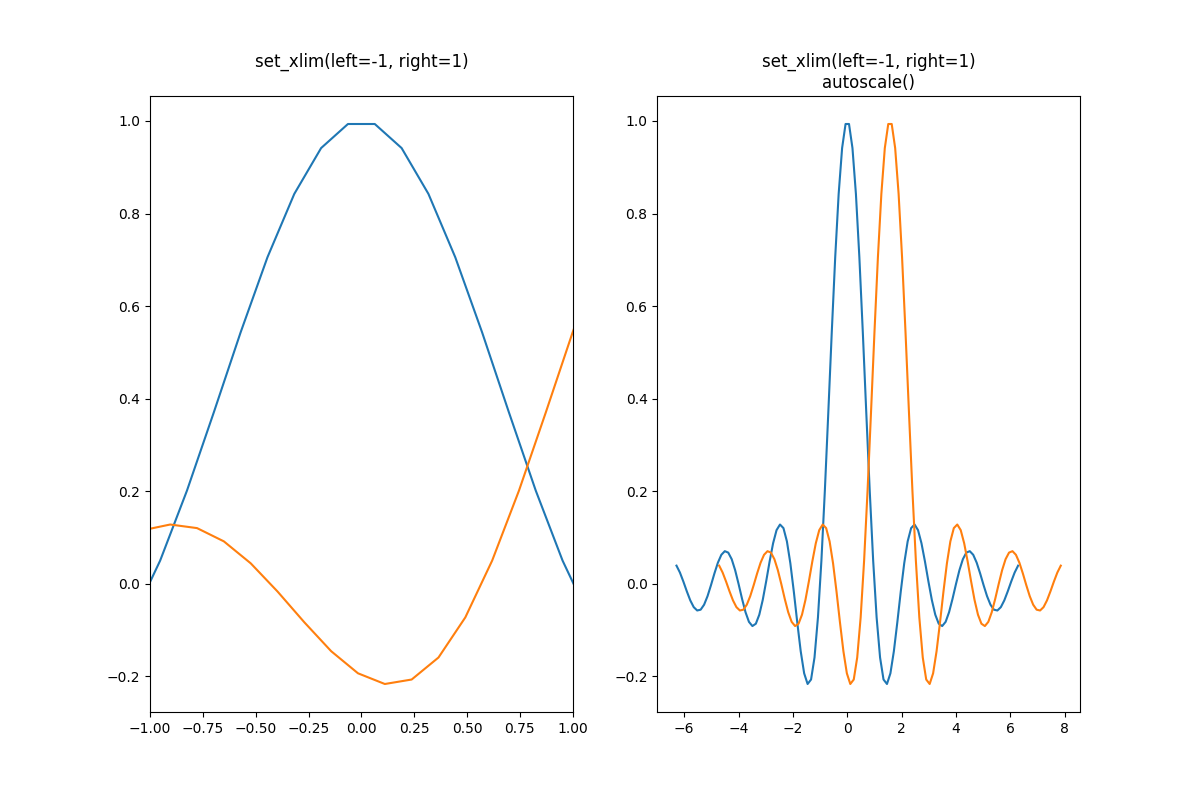Out:

```Text(0.5, 1.0, 'set_xlim(left=-1, right=1)\nautoscale()')
```

We can check that the first plot has autoscale disabled and that the second plot has it enabled again by using `Axes.get_autoscale_on()`:

```print(ax.get_autoscale_on())  # False means disabled
print(ax.get_autoscale_on())  # True means enabled -> recalculated
```

Out:

```False
True
```

Arguments of the autoscale function give us precise control over the process of autoscaling. A combination of arguments `enable`, and `axis` sets the autoscaling feature for the selected axis (or both). The argument `tight` sets the margin of the selected axis to zero. To preserve settings of either `enable` or `tight` you can set the opposite one to None, that way it should not be modified. However, setting `enable` to None and tight to True affects both axes regardless of the `axis` argument.

```fig, ax = plt.subplots()
ax.plot(x, y)
ax.margins(0.2, 0.2)
ax.autoscale(enable=None, axis="x", tight=True)

print(ax.margins())
```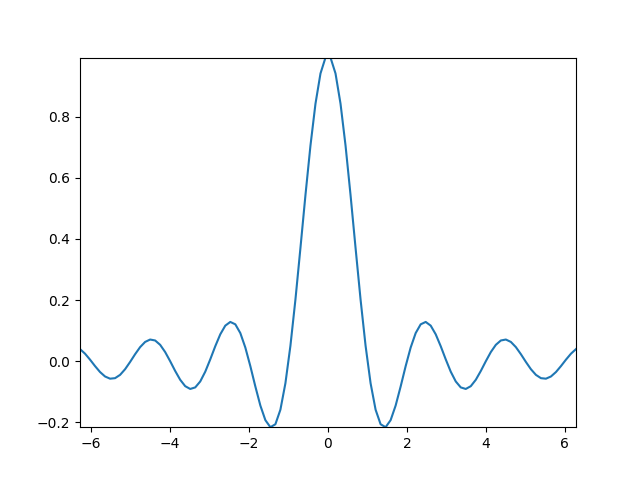Out:

```(0, 0)
```

## Working with collections¶

Autoscale works out of the box for all lines, patches, and images added to the axes. One of the artists that it won't work with is a `Collection`. After adding a collection to the axes, one has to manually trigger the `autoscale_view()` to recalculate axes limits.

```fig, ax = plt.subplots()
collection = mpl.collections.StarPolygonCollection(
5, 0, [250, ],  # five point star, zero angle, size 250px
offsets=np.column_stack([x, y]),  # Set the positions
transOffset=ax.transData,  # Propagate transformations of the Axes
)